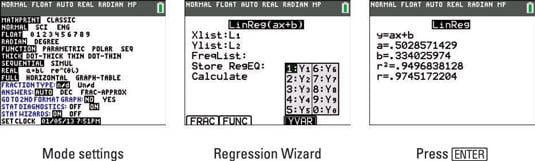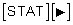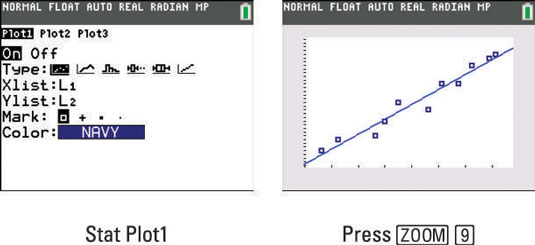##### Ti-84 Plus Graphing Calculator For DummiesRegression modeling is the process of finding a function that approximates the relationship between the two variables in two data lists. The table shows the types of regression models the TI-84 Plus calculator can compute.

Types of Regression Models
TI-Command Model Type Equation
Med-Med Median-median y = ax + b
LinReg(ax+b) Linear y = ax + b
QuadReg Quadratic y = ax2 + bx + c
CubicReg Cubic y = ax3 + bx2 + cx + d
QuartReg Quartic y = ax4 + bx3 + cx2 + dx + e
LinReg(a+bx) Linear y = a + bx
LnReg Logarithmic y = a + b*ln(x)
ExpReg Exponential y = abx
PwrReg Power y = axb
Logistic Logistic y = c/(1 + a*e-bx)
SinReg Sinusoidal y = a*sin(bx + c) + d

To compute a regression model for your two-variable data, follow these steps:

1. If necessary, turn on Diagnostics and put your calculator in Function mode.

When Stat Diagnostics is turned on, the calculator displays the correlation coefficient (r) and the coefficient of determination (r2 or R2) for appropriate regression models (as shown in the third screen). By default, Stat Diagnostics is turned off.

If the regression model is a function that you want to graph, you must first put your calculator in Function mode.

Here's how to turn Stat Diagnostics on and set your calculator to Function mode:

1. Press [MODE].

2. Use the arrow keys to highlight STAT DIAGNOSTICS ON and press [ENTER].

3. Use the arrow keys to highlight FUNCTION and press [ENTER].

The first screen shows this procedure.2. Select a regression model from the Stat CALCULATE menu to activate the Regression Wizard.

To access the Stat CALCULATE menu, pressRepeatedly press the down-arrow key until the number or letter of the desired regression model is highlighted, and press [ENTER] to select that model.

3. Enter the name for the Xlist data and enter the name of the Ylist data.

4. If necessary, enter the name of the frequency list.

5. With your cursor in the Store RegEQ line, enter the name of the function (Y1, ... , Y9, or Y0) in which the regression model is to be stored.

To enter a function name, press a\$ to access the shortcut Y-VAR menu and then enter the number of the function you want, as shown in the second screen.

6. Press [ENTER] on CALCULATE to view the equation of the regression model.

This is illustrated in the third screen. The equation of the regression model is automatically stored in the Y= editor under the name you entered in Step 5.

## Graphing a regression model

Often, it is a good idea to take a look at the scatter plot of your data to determine what type of regression model is best. Here are the steps to graph a scatter plot of your data and the regression model on the same graph:

1. If you haven't already done so, graph your two-variable data in a scatter plot or an xy-line plot.

Set up the scatter plot by pressing [2nd][Y=][ENTER]. See Stat Plot1 in the first screen.2. Press [ZOOM] to see the graph of your data and regression model.

This process is illustrated in the second screen.